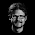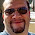## Tuesday, February 16, 2016

### HP Prime: Carmichael Numbers (Update 2/21/2016)

HP Prime:  Carmichael Numbers

Introduction

An integer n is a Carmichael number (1910) when the following holds:

a^n ≡ a mod n

(or for a>0 and n>0)

a^n – integer(a^n/n) * n  = a

For all integers a.

The program ISCARMICHAEL tests whether an integer n qualifies as a Carmichael number based on the Korselt’s Criterion:

1.  n is a positive, composite integer.  That is, n can be factored into a multiplication of prime numbers.
2.  n is square-free.  That is, √n is not an integer.
3. For each prime factor p dividing n (not 1 or n), the following is true: (p-1) divides (n-1) evenly (without fraction).

Update:  I was mistaken about the definition of squarefree.  A squarefree integer are integers that are not divisible by perfect squares (I thought the number was square free itself).  I am to update ISCARMICHAEL this week.  Apologizes and many appreciations to Hank for pointing this out to me.  -  EWS

HP Prime Program ISCARMICHAEL  (Updated 2/21/2016)

EXPORT ISCARMICHAEL(n)
BEGIN
// EWS 2016-02-21
// Thanks: Hank
LOCAL l1,s,flag,k;
LOCAL vect;
flag:=0;

// composite test
IF isprime(n)==1 THEN
RETURN 0; KILL;
END;

// setup
vect:=ifactors(n);
l1:=SIZE(vect);
s:=l1(1);

// square-free number test
FOR k FROM 2 TO s STEP 2 DO
IF vect(k)>1
THEN
flag:=1; BREAK;
END;
END;

// factor test

FOR k FROM 1 TO s STEP 2 DO
IF FP((n-1)/(vect(k)-1))≠0
THEN
flag:=1; BREAK;
END;
END;

// final result
IF flag==0 THEN
RETURN 1;
ELSE
RETURN 0;
END;

END;

Notes:
The commands isprime and ifactors are generated from the CAS-Integer (Options 6 then 1 for isprime; option 3 for ifactors) submenu.  However, I think in order for this program to work properly, the “CAS.” must be deleted and the command should be in all-lowercase.

Examples:

ISCARMICHAEL(561) returns 1.  561 is a Carmichael number.  The prime factors of 561 are 3, 11, 17.

ISCARMICHAEL(778) returns 0, hence 778 is not a Carmichael number.  The prime factors of 778 are 2 and 389.

Source:

Crandall, Richard and Pomerance, Carl.  Prime Numbers: A Computational Perspective  2nd Ed.  Springer: New York.  2005

Eddie

This blog is property of Edward Shore.  2016

1.Thanks for sharing...! I think This blog is very interesting, I hope you feel same like me about my link @ graphing calculator casio online
moderncalculator.com
Moderncalculator

1.Tyler,

I like this! I like the wide variety of applications you have on the page. The site has been Favorited. Thanks!

Eddie

2.Eddie,

Do you think that an article on solving elementary homogenous linear recursions such as x (n + 1) = 2 x (n) might be interesting to your readers? And maybe a program to automate the process?

1.Hank,

This is something I would like to look into. Thank you for the suggestion.

Eddie

2.Eddie,
Looking at a list of Carmichael numbers, I see that 1729 is the third. This is the famous Hardy-Ramanujan number, the smallest positive integer that can be expressed as the sum of two cubes in two different ways: 1^3 + 12^3 and 9^3 + 10^3. The wiki articles on Carmichael Numbers and on the number 1729 are both fascinating.

### Retro Review: Radio Shack EC-2024

Retro Review:  Radio Shack EC-2024 Quick Facts Company:  Radio Shack Years in Production: Around 1994 Original Price: \$24.99 Bat...